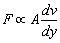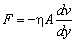# Physics Assignment Help With Stokes law and Terminal velocity

1. Home
2. Physics
3. Matter Properties
4. Terminal velocity

## 9.22 Viscosity and Newton's law of Viscous Force

As a result of large number of experiments Newton found that viscous force F acting on any layer of fluid is directly proportional to its area A and to the velocity gradient (dv/dy) at the layer, i.e.,or… (1)

where h is a constant called coefficient of viscosity or simply viscosity of the fluid. The negative sign shows that viscous force on a liquid layer acts in a direction opposite to the relative velocity of flow of fluid. The equation (1) is known as Newton's law of viscous force.

### Stokes law and Terminal velocity

When a body moves through a fluid, the fluid in contact with the body is dragged with it. This establishes relative motion in fluid layer near the body, due to which viscous force starts operating. The fluid exerts viscous force on the body to oppose its motion. The magnitude of the viscous force depends on the shape and size of the body, its speed and the viscosity of the fluid. Strokes established that if a sphere of radius r moves with velocity v through a fluid of viscosity h, the viscous force opposing the motion of the sphere is

F = 6phru … (4)

This law is called Strokes law.

### Email Based Assignment Help in Viscosity and Newton's law of Viscous Force

We are the leading online Assignment Help provider. Find answers to all of your doubts regarding the Viscosity and Newton’s law of Viscous Force. Assignmenthelp.net provide homework, Assignment Help to the school, college or university level students. Our expert online tutors are available to help you in Viscosity and Newton's law of Viscous Force. Our service is focused on: time delivery, superior quality, creativity and originality.To submit physics Viscosity and Newton's law of Viscous Force assignment click here.

Following are some of the areas in physics Matter Properties which we provide help: